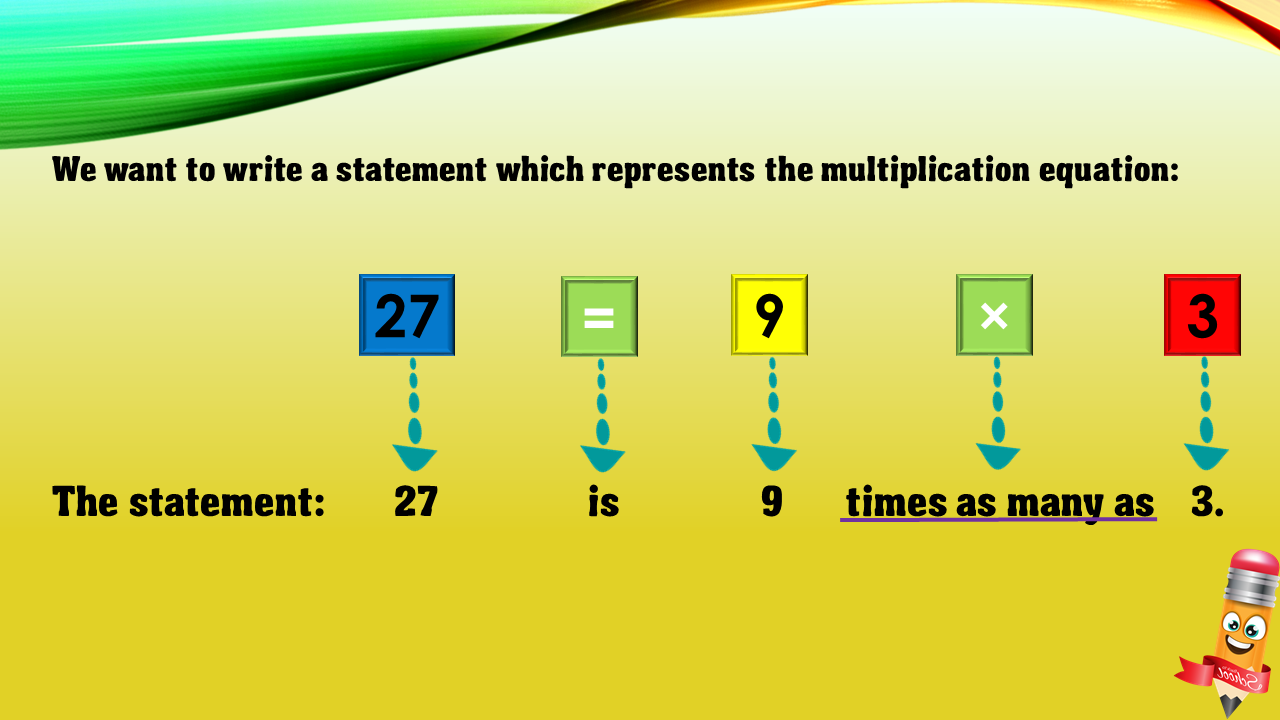1
visibility

Choose one statement which represents the equation: 27 = 9×3 ?

• A

27 is 9 more than 3.

• B

27 times as many as 9 is 3.

• C

9 is 3 less than 27.

• D

27 is 9 times as many as 3.